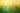## Tips to learn React + Redux in 2019

For quite some time now, I have been implementing applications in React and Redux. Over the last few years, I have written two ebooks about it and released a course platform for learning React and its ecosystem. The course platform is even built in React and Redux . My personal learnings are subjective, but I thought they may help people to…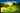## Logistic Regression with Gradient Descent in JavaScript

A couple of my recent articles gave an introduction to machine learning in JavaScript by solving regression problems with linear regression using gradient descent or normal equation . In a regression problem, an algorithm is trained to predict continuous values . It can be housing prices in a specific area based on a feature set such as…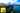## Linear Regression with Normal Equation in JavaScript

A recent article gave an introduction to the field of machine learning in JavaScript by predicting housing prices with gradient descent in a univariate regression problem. It used plain mathematical expressions and thus made use of the unvectorized implementation of gradient descent and the cost function. However, the unvectorized approach doesn…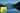## Multivariate Linear Regression, Gradient Descent in JavaScript

A recent article gave an introduction to the field of machine learning in JavaScript by predicting housing prices with gradient descent in a univariate regression problem. It used plain mathematical expressions and thus made use of the unvectorized implementation of gradient descent and the cost function. However, the unvectorized approach doesn…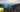## Gradient Descent with Vectorization in JavaScript

A recent article gave an introduction to the field of machine learning in JavaScript by predicting housing prices with gradient descent in a univariate regression problem. It used plain mathematical expressions and thus made use of the unvectorized implementation of gradient descent and the cost function. This article takes it one step further…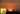## Improving Gradient Descent in JavaScript

A recent article gave an introduction to the field of machine learning in JavaScript by predicting housing prices with gradient descent in a univariate regression problem. There are several improvements to optimize the gradient descent algorithm that should be collected in this article. In general, when dealing with a regression problem, the…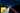## Polynomial Regression and Model Selection

After learning about gradient descent in a linear regression , I was curious about using different kinds of hypothesis functions to improve the result of the algorithm itself. So far, the hypothesis function was only a linear straight line. However, your training set may not have a linear correlation and by staying with a linear model it becomes…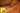## Linear Algebra in JavaScript with Matrix Operations

When I recently started to dive into the topic of machine learning, I had to relearn all the things I have studied about linear algebra, stochastic and calculus at school and university. I took a little refresher on matrix operations (addition, subtraction, multiplication and division) in linear algebra and learned about the different types of…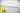## Linear Regression with Gradient Descent in JavaScript

Recently I started to take the Machine Learning course by Andrew Ng on Coursera. So far, it is a blast and I am so keen to apply all my learnings in math from university. Starting right into web development after university, I never had the opportunity to apply those learnings when implementing web applications. Now, it is refreshing to see use…